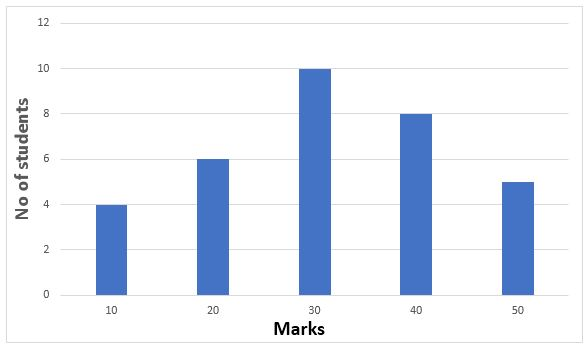## SSC GD Quant Test 14

Instructions

Study the following Histogram and answer the following questions.Question 1

The total number of students involved in the data is

Question 2

The maximum number of students got the marks in the interval of

Question 3

The least number of students got the marks in the interval

Question 4

The ratio of the students obtaining marks in the first and last interval is

Instructions

For the following questions answer them individually

Question 5

In the following number series a wrong number is given. Find out that number.
8, 18, 40, 86, 178, 370, 752